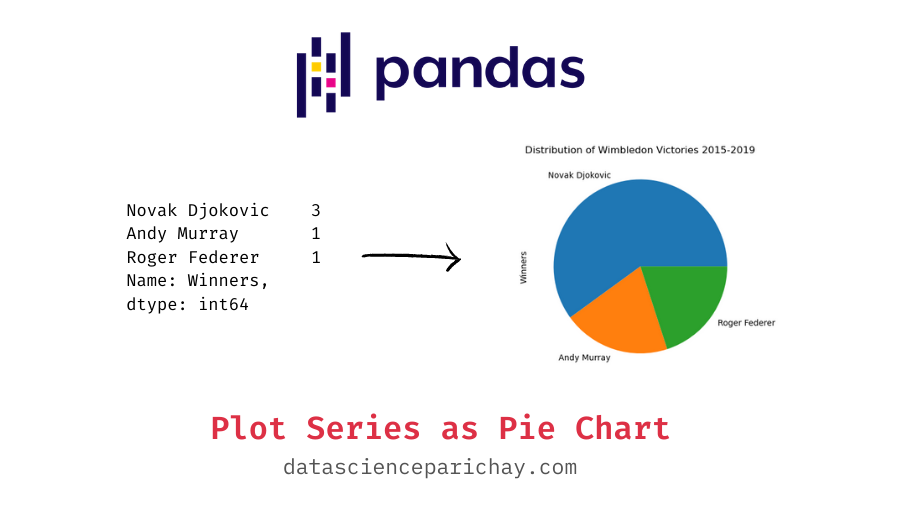# Create a Pie Chart of Pandas Series Values

You can better visualize a pandas series with categorical values via a pie chart of counts. In this tutorial, we will look at how to plot a pie chart of pandas series values.

To plot a pie chart, you first need to create a series of counts of each unique value (use the pandas `value_counts()` function) and then proceed to plot the resulting series of counts as a pie chart using the pandas series `plot()` function.

The `plot()` function plots a line chart of the series values by default but you can specify the type of chart to plot using the `kind` parameter. To plot a pie chart, pass `'pie'` to the `kind` parameter. The following is the syntax:

```# pie chart using pandas series plot()
s.value_counts().plot(kind='pie')```

Here, s is the pandas series with categorical values which is converted to a series of counts using the `value_counts()` function. The pandas series `plot()` function returns a matplotlib axes object to which you can add additional formatting.

Let’s look at some examples of plotting a series values as a pie chart. First, we’ll create a sample pandas series which we will be using throughout this tutorial.

```import pandas as pd

# pandas series Wimbledon winners from 2015 to 2019
wimbledon_winners = pd.Series(index=[2015, 2016, 2017, 2018, 2019],
data=['Novak Djokovic', 'Andy Murray', 'Roger Federer', 'Novak Djokovic', 'Novak Djokovic'],
name='Winners')

# display the series
print(wimbledon_winners)```

Output:

```2015    Novak Djokovic
2016       Andy Murray
2017     Roger Federer
2018    Novak Djokovic
2019    Novak Djokovic
Name: Winners, dtype: object```

You can see the contents of the series object above. We now have a pandas series containing the name of Wimbledon Winners from 2015 to 2019 with the year as its index.

Let’s create a series of counts of championships won by each player during the period using the `value_counts()` function. We’ll be using this series to plot our pie chart.

📚 Data Science Programs By Skill Level

Introductory

Intermediate ⭐⭐⭐

🔎 Find Data Science Programs 👨‍💻 111,889 already enrolled

Disclaimer: Data Science Parichay is reader supported. When you purchase a course through a link on this site, we may earn a small commission at no additional cost to you. Earned commissions help support this website and its team of writers.

```# series of counts
wimbledon_wins_count = wimbledon_winners.value_counts()
# print the counts
print(wimbledon_wins_count)```

Output:

```Novak Djokovic    3
Andy Murray       1
Roger Federer     1
Name: Winners, dtype: int64```

The returned series contains counts of victories by each player in the original series.

To create a pie chart from the series values we’ll pass `kind='pie'` to the pandas series `plot()` function. For example, let’s see its usage on the “wimbledon_wins_count” series created above.

`wimbledon_wins_count.plot(kind='pie')`

Output:

The above pie chart shows the distribution of Wimbledon victories from 2015 to 2019. You can see that Novak Djokovic has won more than half of the championships during that period. Note that the resulting plot is a matplotlib pie chart.

For more on the pandas series plot() function, refer to its documentation.

You can also customize the formatting of the chart. For instance, you can add the axes labels, chart title, change colors and fonts, etc. Since the returned plot is a matplotlib axes object, you can apply any formatting that would work with matplotlib charts.

```# create the pie chart
ax = wimbledon_wins_count.plot(kind='pie')
# set the title
ax.set_title("Distribution of Wimbledon Victories 2015-2019")```

Output:

You can see in the above chart has “Distribution of Wimbledon Victories 2015-2019” as its title.

For more on pie charts and their formatting in matplotlib, refer to our tutorial on matplotlib pie charts.

With this, we come to the end of this tutorial. The code examples and results presented in this tutorial have been implemented in a Jupyter Notebook with a python (version 3.8.3) kernel having pandas version 1.0.5

•